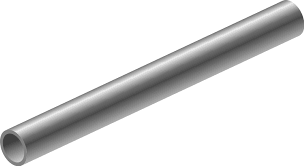# Pipe is that!

Geometry Level 3An iron pipe 20 cm long has exterior diameter equal to 25 cm. If the thickness of the pipe is 1 cm, find the total surface area of the pipe. Write your answer in $\text{cm}^2$.
Note : Take $\pi$ as $\dfrac{22}{7}$.

×